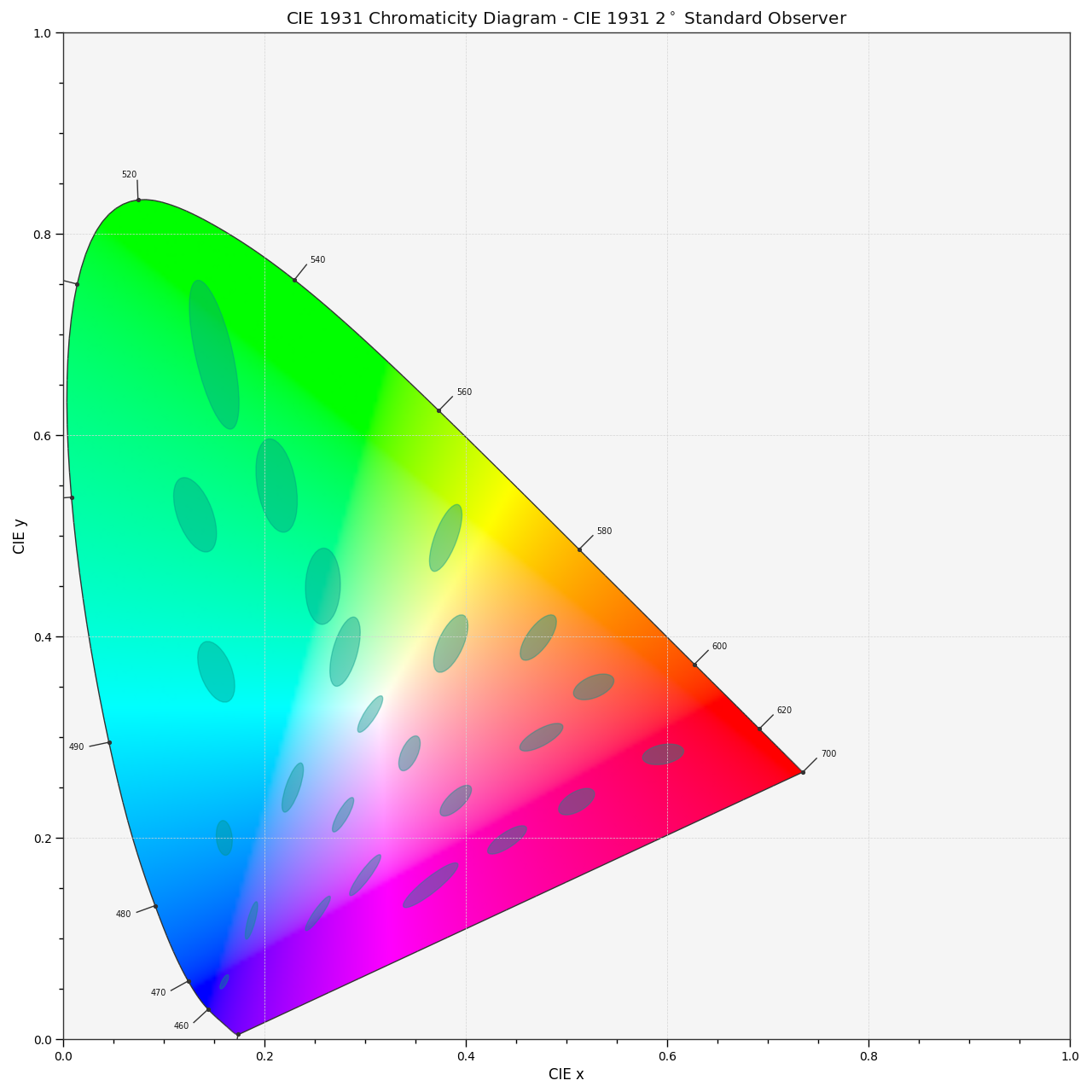colour.plotting.models.plot_ellipses_MacAdam1942_in_chromaticity_diagram(chromaticity_diagram_callable: Callable = plot_chromaticity_diagram, method: Literal['CIE 1931', 'CIE 1960 UCS', 'CIE 1976 UCS'] | str = 'CIE 1931', chromaticity_diagram_clipping: bool = False, ellipse_kwargs: dict | List[dict] | None = None, **kwargs: Any) [source]#

Plot MacAdam (1942) Ellipses (Observer PGN) in the Chromaticity Diagram according to given method.

Parameters:
• chromaticity_diagram_callable (Callable) – Callable responsible for drawing the Chromaticity Diagram.

• method (Literal['CIE 1931', 'CIE 1960 UCS', 'CIE 1976 UCS'] | str) – Chromaticity Diagram method.

• chromaticity_diagram_clipping (bool) – Whether to clip the Chromaticity Diagram colours with the ellipses.

• ellipse_kwargs (dict | List[dict] | None) – Parameters for the `Ellipse` class, `ellipse_kwargs` can be either a single dictionary applied to all the ellipses with same settings or a sequence of dictionaries with different settings for each ellipse.

• kwargs (Any) – {`colour.plotting.artist()`, `colour.plotting.diagrams.plot_chromaticity_diagram()`, `colour.plotting.render()`}, See the documentation of the previously listed definitions.

Returns:

Current figure and axes.

Return type:

`tuple`

Examples

```>>> plot_ellipses_MacAdam1942_in_chromaticity_diagram()
...
(<Figure size ... with 1 Axes>, <...Axes...>)
```